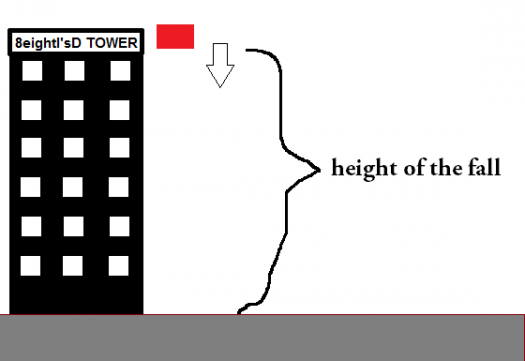# Engineering Mechanics - dynamics

2 posts / 0 new
Santanina Gonza...Engineering Mechanics - dynamics

A hollow block is accidentally dropped by a laborer working in a high rise building. four seconds after it strikes the ground, an explosion like sound was heard by the laborer. the velocity of sound is 320 minutes per seconds.

1. In what storey number of the building the laborer is located if the height per storey is
3 meters?
2. What is the time of the hollow block to reach the ground?
3. what is the time of sound to travel the distance equal to the position (height) of the
laborer?

fitzmerl duron#1

To get the storey number of the laborer when he droped the hollow block,we need to find the height of the laborer from the ground.

$distance = (velocity)(time)$

Then...

$$height \space of \space the \space laborer = (speed \space of \space sound)(time \space taken \space before \space the \space laborer \space heard \space the \space sound)$$ $$d =\left (320 \frac{meters}{second}\right)(4 \space second)$$ $$d = 1 280 \space meters$$

Storey number = $(1280\space meters)\left(\frac{1 \space storey}{3 \space meters}\right)$
Storey number = $426 \space storeys$

Therefore, the laborer was at $426$th floor when he dropped the hollow block

#2

To get the time of the hollow block to reach the ground, do this....

Using the kinematic equation $v_f^2 = v_i^2 + 2ad$, where:

$v_f$ = final velocity
$v_i$ = initial velocity
$a$ = acceleration
$d$ = distance between two places

Then...

$$v_f^2 = v_i^2 + 2ad$$ $$v_f^2 =\left (0 \space \frac{meters}{second}\right)^2 + 2\left(9.8 \space \frac{meters}{second^2}\right)(120 \space meters)$$ $$v_f = 158 \frac{meters}{second}$$

We set the initial velocity as zero because the laborer is holding it before the hollow block fell.

To finally get the time needed by the hollow block to reach the ground, use another kinematic equation $d = v_f t + 0.5at^2$

Then...

$$d = v_f t + 0.5at^2$$ $$1 280 \space meters = \left(158\space \frac{meters}{second}\right)(t) + 0.5\left(9.8 \space \frac{meters}{second}\right)(t)^2$$

Recognizing that the equation above is a quadratic equation, we reaarange the equation now, getting...

$$4.9t^2 + 158t - 1280 = 0$$

Solving the unknown, it was found out that $t_1 = 6.7$ and $t_2 = - 38.9$. The negative answer is absurd, so the real answer would be $t = 6.7$

Therefore, the time needed by the hollow block to reach the ground would be $6.7$ seconds

#3

To get the time of sound to travel the distance equal to the position (height) of the laborer, just use the basic formula $d = vt$

Then...

$$d = vt$$ $$1280 \space meters = \left(320\space \frac{meters}{second}\right) (t)$$ $$t = 4 \space seconds$$

Therefore...the time of sound to travel the distance equal to the position (height) of the laborer would be $4$ seconds...

Alternate ways of solving are encouraged.....I believe it is correct. Did I get it right?

Subscribe to MATHalino on